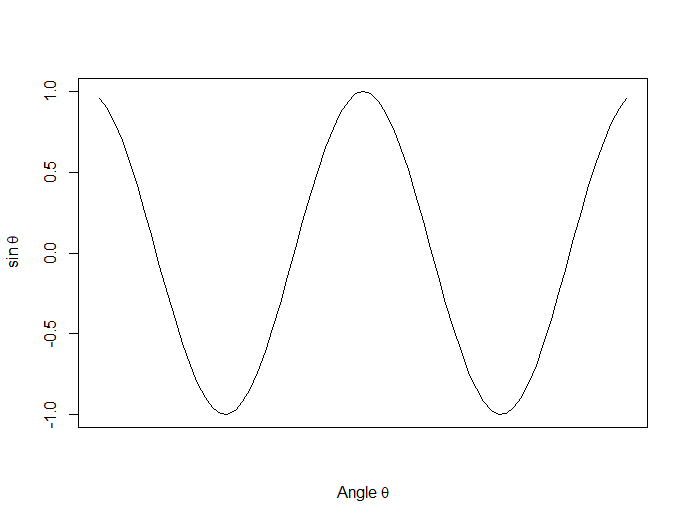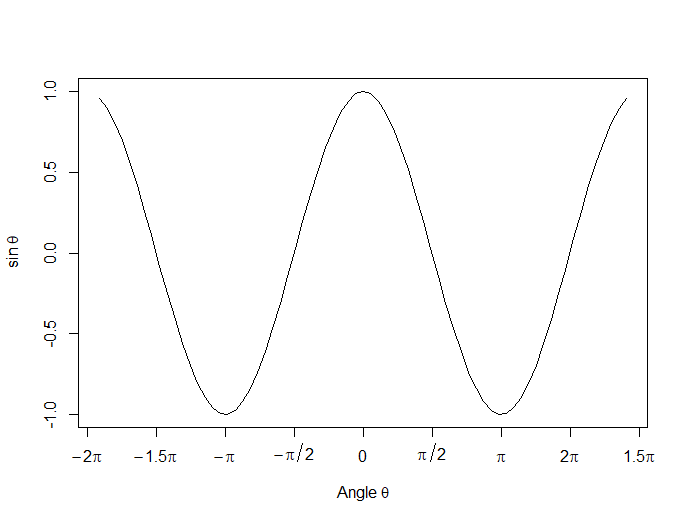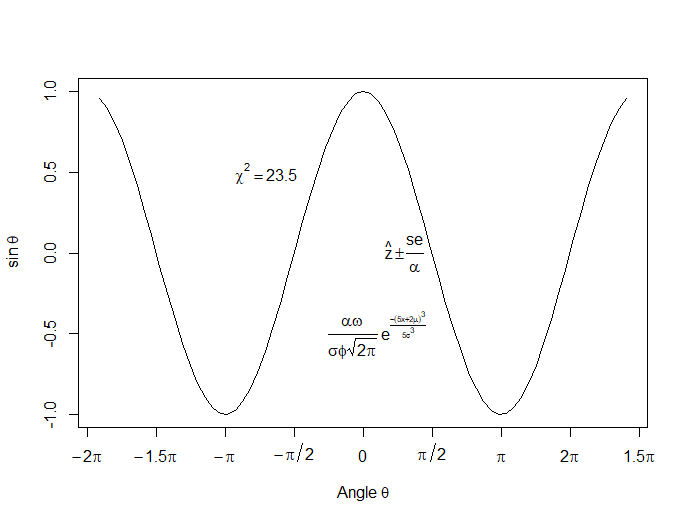# Graphing Non-Linear Mathematical Expressions in R

by David Lillis, Ph.D.In Part 20 of this series, let’s see how to create mathematical expressions for your graph in R.  We’ll use an example of graphing a cosine curve, along with relevant Greek letters as the axis label, and printing the equation right on the graph.

Mathematical expressions, like sine or exponential curves on graphs are made possible through `expression(paste())` and `substitute()`.

If you need mathematical symbols as axis labels, switch off the default axes and include Greek symbols by writing them out in English. You can create fractions through the `frac()` command. Note how we obtain the plus or minus sign through the syntax: `%+-%`

Here is a nice example. Let’s create a set of 71 values from – 6 to + 6. These values are the horizontal axis values.

`x <- seq(-6, 6, len = 71)`

Now we plot a cosine function using a continuous curve (using `type="l"`) while suppressing the x axis using the syntax: `xaxt="n"`

```plot(x, cos(x),type="l",xaxt="n", xlab=expression(paste("Angle ",theta)), ylab=expression("sin "*theta)) ```
. . . where we have inserted relevant mathematical text for the axis labels using `expression(paste())`. Here is the graph so far:Now we create a horizontal axis to our own specifications, including relevant labels:

```axis(1, at = c(-2*pi, -1.5*pi, -pi, -pi/2, 0, pi/2, pi, 1.5*pi, 2*pi), lab = expression(-2*pi, -1.5*pi, -pi, -pi/2, 0, pi/2, pi, 2*pi, 1.5*pi))```Let’s put in some mathematical expressions, centered appropriately. The first argument within each `text()` function gives the value along the horizontal axis about which the text will be centered.

`text(-0.7*pi,0.5,substitute(chi^2=="23.5"))`

``` text(0.1*pi, -0.5, expression(paste(frac(alpha*omega, sigma*phi*sqrt(2*pi)), " ", e^{frac(-(5*x+2*mu)^3, 5*sigma^3)}))) ```

```text(0.3*pi,0,expression(hat(z) %+-% frac(se, alpha))) ```

Here is our graph, complete with mathematical expressions:About the Author: David Lillis has taught R to many researchers and statisticians. His company, Sigma Statistics and Research Limited, provides both on-line instruction and face-to-face workshops on R, and coding services in R. David holds a doctorate in applied statistics.

1.anand says